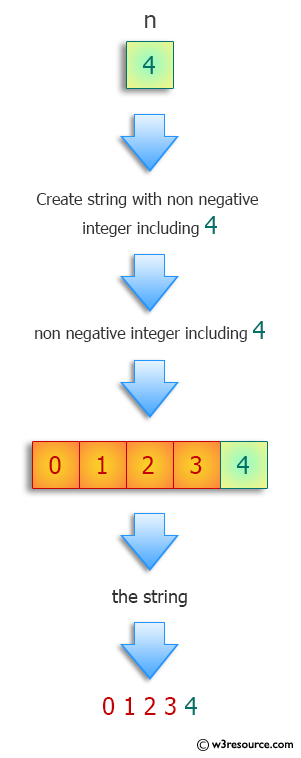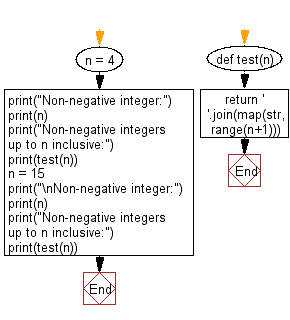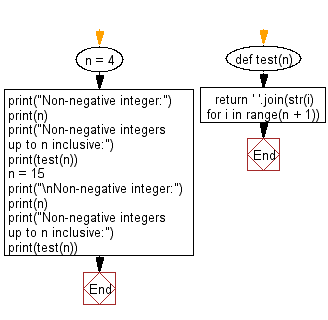﻿ Python: Find a string consisting of the non-negative integers up to n inclusive - w3resource# Python: Find a string consisting of the non-negative integers up to n inclusive

## Python Programming Puzzles: Exercise-17 with Solution

Write a Python program to create string consisting of the non-negative integers up to n inclusive.

```Input:
4
Output:
0 1 2 3 4

Input:
15
Output:
0 1 2 3 4 5 6 7 8 9 10 11 12 13 14 15
```

Pictorial Presentation:Sample Solution-1:

Python Code:

``````def test(n):
return ' '.join(map(str,range(n+1)))
n = 4
print("Non-negative integer:")
print(n)
print("Non-negative integers up to n inclusive:")
print(test(n))
n = 15
print("\nNon-negative integer:")
print(n)
print("Non-negative integers up to n inclusive:")
print(test(n))
``````

Sample Output:

```Non-negative integer:
4
Non-negative integers up to n inclusive:
0 1 2 3 4

Non-negative integer:
15
Non-negative integers up to n inclusive:
0 1 2 3 4 5 6 7 8 9 10 11 12 13 14 15
```

Flowchart:## Visualize Python code execution:

The following tool visualize what the computer is doing step-by-step as it executes the said program:

Sample Solution-2:

Python Code:

``````def test(n):
return ' '.join(str(i) for i in range(n + 1))
n = 4
print("Non-negative integer:")
print(n)
print("Non-negative integers up to n inclusive:")
print(test(n))
n = 15
print("\nNon-negative integer:")
print(n)
print("Non-negative integers up to n inclusive:")
print(test(n))
``````

Sample Output:

```Non-negative integer:
4
Non-negative integers up to n inclusive:
0 1 2 3 4

Non-negative integer:
15
Non-negative integers up to n inclusive:
0 1 2 3 4 5 6 7 8 9 10 11 12 13 14 15
```

Flowchart:## Visualize Python code execution:

The following tool visualize what the computer is doing step-by-step as it executes the said program:

Python Code Editor :

Have another way to solve this solution? Contribute your code (and comments) through Disqus.

What is the difficulty level of this exercise?

Test your Programming skills with w3resource's quiz.

﻿

## Python: Tips of the Day

Clamps num within the inclusive range specified by the boundary values x and y:

Example:

```def tips_clamp_num(num,x,y):
return max(min(num, max(x, y)), min(x, y))
print(tips_clamp_num(2, 4, 6))
print(tips_clamp_num(1, -1, -6))
```

Output:

```4
-1
```